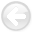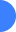Tomographic full waveform inversion (TFWI) by combining full waveform inversion with wave-equation migration velocity analysisNext: Introduction Up: Reproducible Documents

# Tomographic full waveform inversion (TFWI) by combining full waveform inversion with wave-equation migration velocity analysis

Biondo Biondi and Ali Almomin

### Abstract:

By extending the velocity-model domain to subsurface offsets we solve the local-minima problem of data-fitting waveform inversion. We then regularize the extended-model data-fitting inversion with the addition of an image-focusing term to the objective function, therefore achieving robust global convergence of the waveform inversion problem. The method shares with full waveform inversion the advantage of simultaneously solving for all the wavelengths of the model, but it also has the global convergence characteristics of wave-equation migration velocity analysis. Numerical implementation of the proposed inversion method requires the solution of an extended wave-equation where velocity is a convolutional, instead of scalar, operator. The resulting method is therefore computationally intensive, but it can be easily tested in 2D. A simple example with a Gaussian velocity anomaly illustrates how the reflections from the anomaly recorded in the low-frequency components of the data increase the spatial resolution of the final inversion results. Numerical tests performed on synthetic data from a modified Marmousi model demonstrate the global convergence as well the high-resolution potential of the method.Tomographic full waveform inversion (TFWI) by combining full waveform inversion with wave-equation migration velocity analysisNext: Introduction Up: Reproducible Documents

2012-05-10